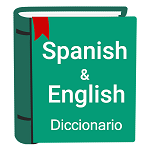### D - Dictionary search

##### Languages: C, C++, Java, Python, ... (details)

Fito is currently learning English. Last week he learned that in English, pretty much like in Spanish, there are some prefixes that can be used to form new words. For example, consider the words "treat" and "understand". If we append "mis" in front of them, we get "mistreat", which means treat someone/something badly, and "misunderstand", which means understand something wrong. In Spanish, this will be equivalent to adding the prefix "mal" in front of the words "tratar" (treat) and "entender" (understand), the results would be "maltratar" which means mistreat and "malentender", which means misunderstand.

He realized, that usually, this kind of prefix in English have an equivalent in Spanish. In this case, the equivalent to "mis" in English would be "mal" in Spanish. Another example is "un" (English)  / "des" (Spanish):

• do -> undo / hacer -> deshacer
• fold -> unfold / plegar -> desplegar

There are many more prefixes: "dis", "pre", "con", "com", "uni", "inter", "sub" , "re"..., etc, but sometimes Fito is not sure about the equivalent prefix in Spanish. Therefore he wants to search in a Dictionary for some pairs of prefixes, how many words do exist such that the English word starts with the first prefix and the word in Spanish (with the same mining) starts with the second prefix.Your task is to help a Fito implementing a program that computes this automatically. More formally, you are given a list of $N$ pairs of words, where each pair consists of a word in English and its equivalent word in Spanish. Next, you are given $Q$ queries consisting of two prefixes. For each query, your task is to write a program which outputs the number of pairs of words in the given list, such that the English word starts with the first prefix and the Spanish word starts with the second prefix.

#### Input

The first line contains two integers $N$ and $Q$, where $N$ ($1 \leq N \leq 10^5$) is the number of words in the list, and $Q$ ($1 \leq Q \leq 10^5$) is the number of queries. The $i$-th line of the following $N$ lines contains two strings $a_i$ and $b_i$. The $j$-th of the following $Q$ lines contains two strings $p_i$ and $q_i$, which form the query.

The input satisfies the following conditions:
• All the strings in the input are non-empty and consist only of lowercase English letters.
• The words can have any length (they might not be real English or Spanish words).
• The total length of the input strings does not exceed $5000000$.
• Words in the given list are unique: if $i \neq j$ then $a_i \neq a_j$  and $b_i \neq b_j$.

#### Output

For each query $(p_j, q_j)$, print in a single line the number of pairs of words $(a,\ b)$ in the given list, such that $p_j$ is a prefix of $a_i$ and $q_i$ is a prefix of $b_i$.

#### Sample test(s)

Input
12 6 mistreat maltratar misunderstand malentender undo deshacer unfold desplegar neglect descuidar decompose descomponer disappear desaparecer compose componer compare comparar combine combinar share compartir collaborate colaborar mis mal un des dis des com com co co no match
Output
2 2 1 3 4 0
Input
5 5 a b aa bb aaa bbb aaaa bbbb aaaaa bbbbb a b aa bb aaa bbb aaaa bbbb aaaaa bbbbb
Output
5 4 3 2 1